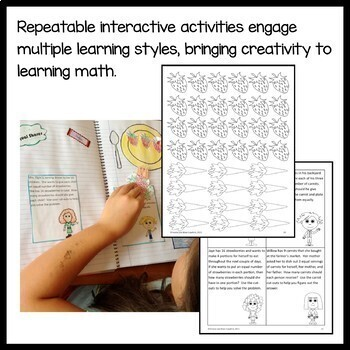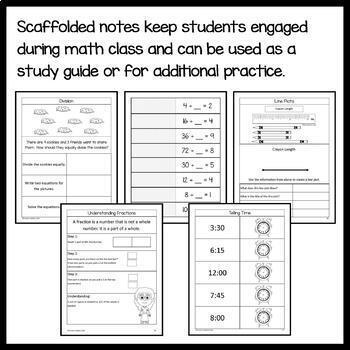DID YOU KNOW:
Seamlessly assign resources as digital activities

Learn how in 5 minutes with a tutorial resource. Try it Now

Learn More2nd - 4th, Homeschool
Subjects
Standards
Resource Type
Formats Included
• Zip
Pages
517 pages

#### Also included in

1. Interactive Notebook Bundle for Third Grade - 1,700+ pages - answer keys included! This bundle contains four interactive notebooks for third grade, supplying everything you need to keep students engaged in interactive learning for a whole year! Great for distance learning!Interactive Notebooks Inclu
\$99.95
\$159.80
Save \$59.85
2. Interactive Math Notebook Bundle for 1st grade, 2nd grade, 3rd grade, 4th grade and 5th grade - 2,335 pages! Scaffolded Notes, 80 interactive printables and 40 Google Slides are also included for each grade level. ANSWER KEYS ARE INCLUDED! This bundle of interactive notebooks contains five interac
\$119.85
\$199.75
Save \$79.90
3. Interactive Math Notebook for Third Grade with Scaffolded Guided Notes + Google Slides - 975 pages! ANSWER KEYS ARE INCLUDED! This huge bundle has everything you need for a full year of teaching 3rd grade math. 105 pages of scaffolded notes have been added including answer keys! Also included are
\$81.86
\$90.95
Save \$9.09

### Description

Interactive Math Notebook for Third Grade - 517 pages - answer keys included! Great for distance learning!

This huge bundle has everything you need for a full year of teaching 3rd grade math:

• A 286-page hands-on interactive math notebook including answer keys
• 105 pages of scaffolded notes including answer keys
• 86 pages of interactive printables including answer keys
• 40 Google Slides of the interactive printables for online teaching or distance learning

Interactive Math Notebook

This math notebook is completely hands-on and interactive. Each chapter includes:

• A divider for the standard that is covered in the chapter
• A hands-on activity for students to put in their math notebooks and use for skills practice and review
• One or more printables you can use as assessments, additional skills practice, morning work, or homework
• A page of graphics that your students can color, cut out, and paste into their math notebooks
• Pictures of children using this notebook to give you and your students ideas about how to set up your own math notebooks

Scaffolded Notes

Included in this product are 105 pages of scaffolded notes (guided notes) and activities. Students will be more engaged in your lessons when using these notes to help guide their learning. Answer keys are provided.

Interactive Printables

These interactive printables can be used as homework, bell work, morning work, sub work, or for skills practice and reinforcement. Great for digital learning! Includes:

• 40 interactive printables
• 40 Google Slides of the interactive printables

All Common Core State Standards (CCSS) for Third Grade Mathematics are covered in this book.

1. Multiplication Using Arrays
2. Understanding Division
3. Multiplication and Division Word Problems
4. Elements of Multiplication and Division
5. Properties of Multiplication
6. Relating Division to Multiplication
7. Multiplication and Division Within 100
8. Two-step Word Problems
9. Patterns of Arithmetic
10. Rounding Numbers
11. Addition and Subtraction Within 1000 Multiplying One-digit Numbers by Multiples of 10
12. Properties of Fractions
13. Representing Fractions on a Number Line
14. Comparing Fractions
15. Telling Time
16. Measurements
17. Bar Graphs
18. Collecting Data and Creating Line Plots
19. Understanding Area
20. Measuring Area
21. Finding the Area of a Rectangle
22. Perimeters
23. Categories of Shapes
24. Expressing Parts of Shapes as Fractions

Check out my Third Grade Interactive Notebook Bundle, including interactive notebooks for math, reading, writing, and grammar at a huge discount!

Interactive math notebooks for other grade levels:

All graphics are originals and created by myself.

Thank you for visiting my store,

Yvonne Crawford

Total Pages
517 pages
Included
Teaching Duration
1 Year
Report this Resource to TpT
Reported resources will be reviewed by our team. Report this resource to let us know if this resource violates TpT’s content guidelines.

### Standards

to see state-specific standards (only available in the US).
Look for and express regularity in repeated reasoning. Mathematically proficient students notice if calculations are repeated, and look both for general methods and for shortcuts. Upper elementary students might notice when dividing 25 by 11 that they are repeating the same calculations over and over again, and conclude they have a repeating decimal. By paying attention to the calculation of slope as they repeatedly check whether points are on the line through (1, 2) with slope 3, middle school students might abstract the equation (𝑦 – 2)/(𝑥 – 1) = 3. Noticing the regularity in the way terms cancel when expanding (𝑥 – 1)(𝑥 + 1), (𝑥 – 1)(𝑥² + 𝑥 + 1), and (𝑥 – 1)(𝑥³ + 𝑥² + 𝑥 + 1) might lead them to the general formula for the sum of a geometric series. As they work to solve a problem, mathematically proficient students maintain oversight of the process, while attending to the details. They continually evaluate the reasonableness of their intermediate results.
Look for and make use of structure. Mathematically proficient students look closely to discern a pattern or structure. Young students, for example, might notice that three and seven more is the same amount as seven and three more, or they may sort a collection of shapes according to how many sides the shapes have. Later, students will see 7 × 8 equals the well remembered 7 × 5 + 7 × 3, in preparation for learning about the distributive property. In the expression 𝑥² + 9𝑥 + 14, older students can see the 14 as 2 × 7 and the 9 as 2 + 7. They recognize the significance of an existing line in a geometric figure and can use the strategy of drawing an auxiliary line for solving problems. They also can step back for an overview and shift perspective. They can see complicated things, such as some algebraic expressions, as single objects or as being composed of several objects. For example, they can see 5 – 3(𝑥 – 𝑦)² as 5 minus a positive number times a square and use that to realize that its value cannot be more than 5 for any real numbers 𝑥 and 𝑦.
Attend to precision. Mathematically proficient students try to communicate precisely to others. They try to use clear definitions in discussion with others and in their own reasoning. They state the meaning of the symbols they choose, including using the equal sign consistently and appropriately. They are careful about specifying units of measure, and labeling axes to clarify the correspondence with quantities in a problem. They calculate accurately and efficiently, express numerical answers with a degree of precision appropriate for the problem context. In the elementary grades, students give carefully formulated explanations to each other. By the time they reach high school they have learned to examine claims and make explicit use of definitions.
Use appropriate tools strategically. Mathematically proficient students consider the available tools when solving a mathematical problem. These tools might include pencil and paper, concrete models, a ruler, a protractor, a calculator, a spreadsheet, a computer algebra system, a statistical package, or dynamic geometry software. Proficient students are sufficiently familiar with tools appropriate for their grade or course to make sound decisions about when each of these tools might be helpful, recognizing both the insight to be gained and their limitations. For example, mathematically proficient high school students analyze graphs of functions and solutions generated using a graphing calculator. They detect possible errors by strategically using estimation and other mathematical knowledge. When making mathematical models, they know that technology can enable them to visualize the results of varying assumptions, explore consequences, and compare predictions with data. Mathematically proficient students at various grade levels are able to identify relevant external mathematical resources, such as digital content located on a website, and use them to pose or solve problems. They are able to use technological tools to explore and deepen their understanding of concepts.
Model with mathematics. Mathematically proficient students can apply the mathematics they know to solve problems arising in everyday life, society, and the workplace. In early grades, this might be as simple as writing an addition equation to describe a situation. In middle grades, a student might apply proportional reasoning to plan a school event or analyze a problem in the community. By high school, a student might use geometry to solve a design problem or use a function to describe how one quantity of interest depends on another. Mathematically proficient students who can apply what they know are comfortable making assumptions and approximations to simplify a complicated situation, realizing that these may need revision later. They are able to identify important quantities in a practical situation and map their relationships using such tools as diagrams, two-way tables, graphs, flowcharts and formulas. They can analyze those relationships mathematically to draw conclusions. They routinely interpret their mathematical results in the context of the situation and reflect on whether the results make sense, possibly improving the model if it has not served its purpose.